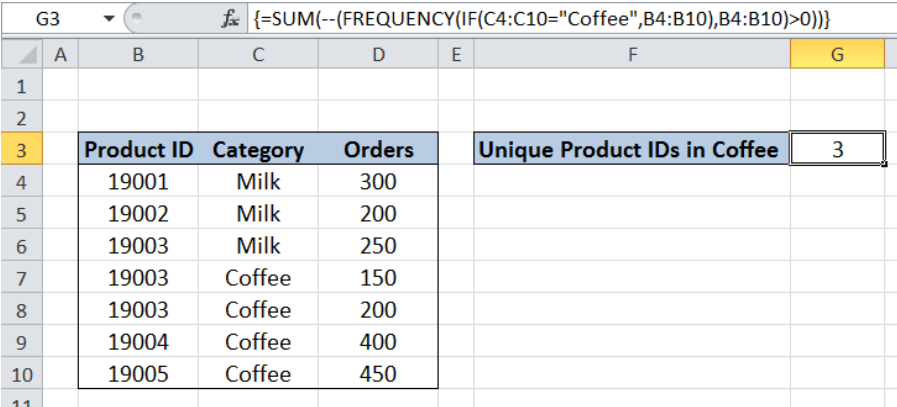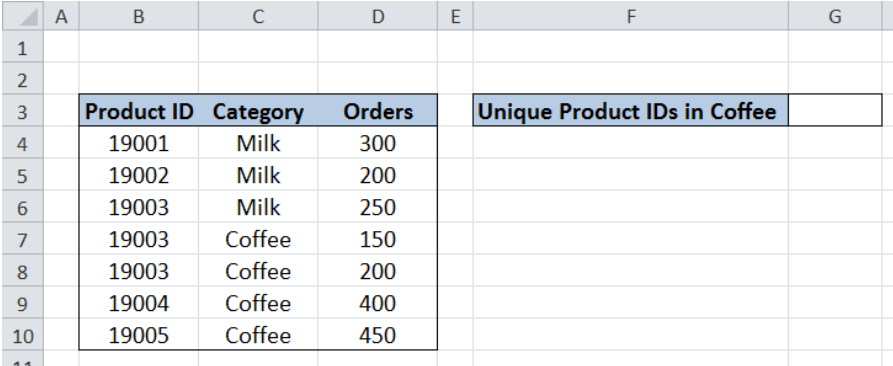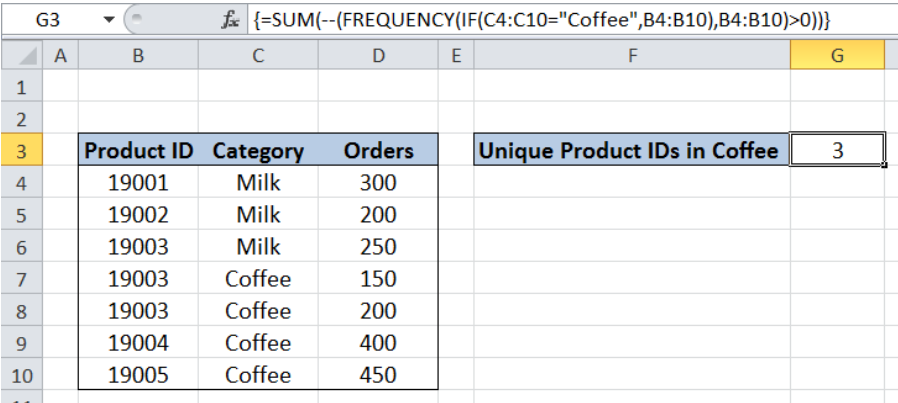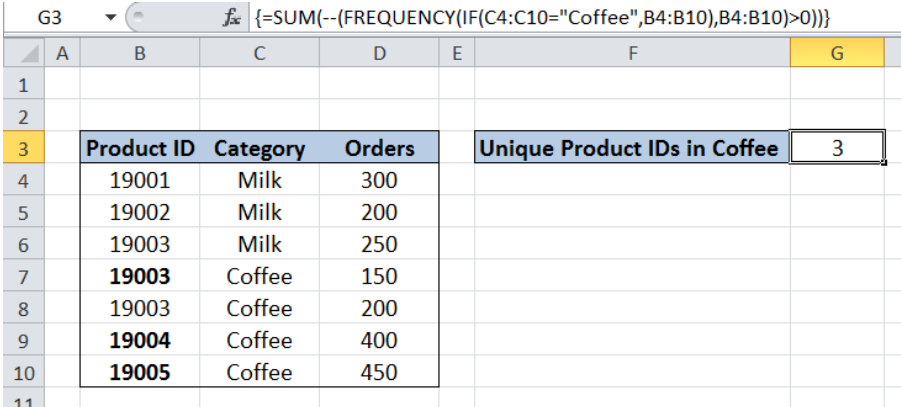Get instant live expert help with Excel or Google Sheets“My Excelchat expert helped me in less than 20 minutes, saving me what would have been 5 hours of work!”

#### Post your problem and you’ll get Expert help in seconds.

Your message must be at least 40 characters
Our professional Expert are available now. Your privacy is guaranteed.

# How to Count Unique Numeric Values with Criteria

While working with Excel, we are able to count unique numeric values in a data set by using the SUM, FREQUENCY and IF functions, with some help using the double negative “”.  This step by step tutorial will assist all levels of Excel users in counting unique numeric values based on a criteria.Figure 1. Final result: Count unique numeric values with criteria

Final formula:  `{=SUM(--(FREQUENCY(IF(C4:C10="Coffee",B4:B10),B4:B10)>0))}`

## Syntax of SUM Function

`=SUM(number1,[number2],...])`

• number1 – any number, array or cell reference whose values we want to add
• Only number1 is required; succeeding numbers are optional

## Syntax of the FREQUENCY function

FREQUENCY determines how often values occur within a range, and returns a vertical array of numbers. Since FREQUENCY returns an array, it must be entered as an array formula.

FREQUENCY automatically returns zero for numbers that appear more than once in the data array

`=FREQUENCY(data_array, bins_array)`

• data_array  – an array or reference to a set of values whose frequencies we want to count
• bins_array – an array or reference to intervals specifying how we want to group the values in data_array
• FREQUENCY ignores blank cells

## Syntax of IF Function

IF function evaluates a given logical test and returns a TRUE or a FALSE

`=IF(logical_test, [value_if_true], [value_if_false])`

• The arguments “value_if_true” and “value_if_false” are optional.  If left blank, the function will return TRUE if the logical test is met, and FALSE if otherwise.

## Setting up Our Data

Our table contains three columns: Product ID (column B), Category (column C) and Orders (column D).  We want to determine the number of unique Product IDs in the category “Coffee” and record it in cell G3.Figure 2. Sample data to count unique numeric values with criteria

## Count unique Product IDs in Coffee

In order to count unique Product IDs in Coffee, we follow these steps:

Step 1.  Select cell G3

Step 2.  Enter the formula: `=SUM(--(FREQUENCY(IF(C4:C10="Coffee",B4:B10),B4:B10)>0))`

Step 3:  Press Ctrl + Shift + Enter

FREQUENCY returns an array so our formula needs to be entered as an array formula by pressing Ctrl + Shift + Enter.Figure 3. Entering the formula using SUM, FREQUENCY and IF

`=SUM(--(FREQUENCY(IF(C4:C10="Coffee",B4:B10),B4:B10)>0))`

Our data_array for FREQUENCY is the IF function `IF(C4:C10="Coffee",B4:B10)`. IF returns the value in column B if the category in column C is “Coffee”.  The result is an array like this:

`{FALSE;FALSE;FALSE;19003;19003;19004;19005}`

The FREQUENCY function then returns the number of times a value occurs in the array.  However, it automatically returns a zero for values occurring more than once. The resulting array is:

`{0;0;0;2;0;1;1}`

The array is then tested to be greater than zero.

`{FALSE;FALSE;FALSE;TRUE;FALSE;TRUE;TRUE}`

The double negative sign “–” converts the values TRUE and FALSE into 1 and 0, respectively.

The array becomes: `{0;0;0;1;0;1;1}`

Finally, the result in cell G3 is the sum of the values in the array which is 3.  There are three unique Product IDs with the category “Coffee”: 19003, 19004 and 19005.Figure 4. Output: Count unique numeric values with criteria

Most of the time, the problem you will need to solve will be more complex than a simple application of a formula or function. If you want to save hours of research and frustration, try our live Excelchat service! Our Excel Experts are available 24/7 to answer any Excel question you may have. We guarantee a connection within 30 seconds and a customized solution within 20 minutes.

### Did this post not answer your question? Get a solution from connecting with the expert.Another blog reader asked this question today on Excelchat:
Solution examplesI am trying to have a tab change color when the Sum of a row = 0. I am using code on the tab but it appears to be confused because the value of the given cell is not 0 it is "=SUM(G4:G17)"
Solved by S. F. in 41 minsCould you help me by explaining the IF function?
Solved by G. L. in 24 minsI have a question about Conditional Formatting. =IF('Sheet1'!A1="X",IF('Sheet1'!B1="Y", TRUE, FALSE),FALSE) the set the cell background to red. I would like to make it so that if Sheet1'!B1="Z" I set the back to blue. How can I achieve this.
Solved by B. F. in 39 minsI need to find out if the 2018 yearly sales goals were met if the yearly sales were \$25,000 or more using an IF logical function and to set the formula to return a value of YES if met, and NO if not
Solved by A. A. in 18 minsI filtered data in column A (it is labeled ID #) of a data set. On my next worksheet, column A is also the ID #, but it is a different data set. I want to filter the ID #'s the same for the two sheets, but how do I do that being that I am working with two different sets of data?
Solved by V. H. in 22 mins## Subscribe to Excelchat.coAnother blog reader asked this question today on Excelchat: